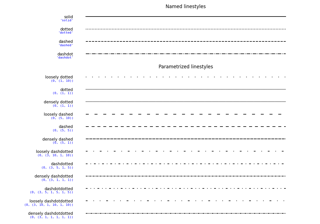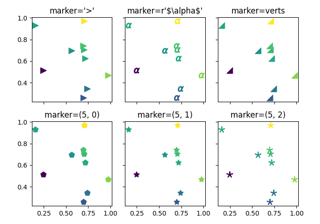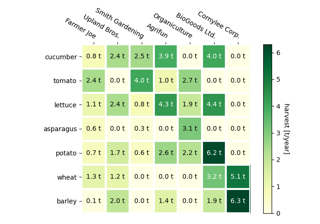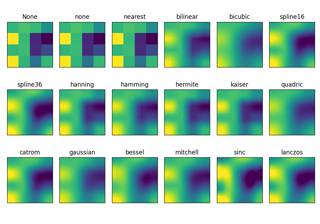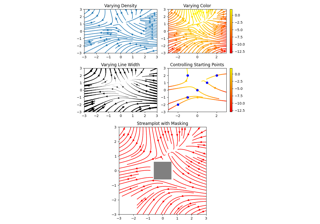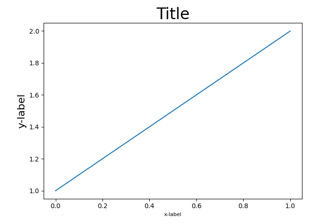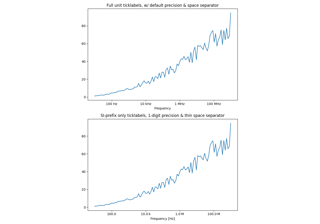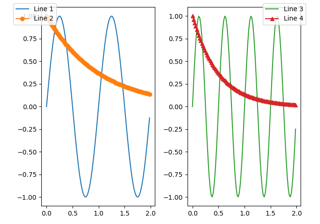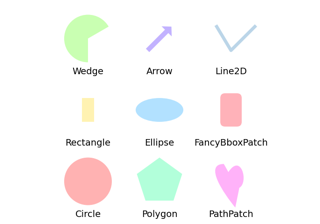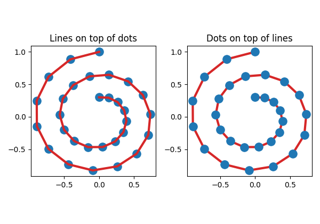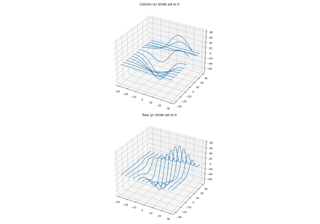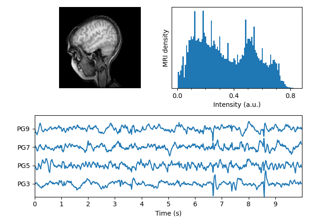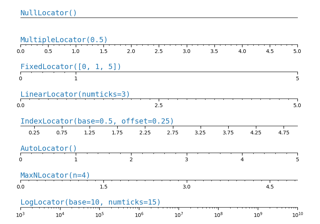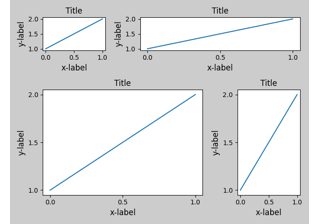# matplotlib.pyplot.tight_layout¶

matplotlib.pyplot.tight_layout(*, pad=1.08, h_pad=None, w_pad=None, rect=None)[source]

Parameters: padfloat, default: 1.08Padding between the figure edge and the edges of subplots, as a fraction of the font size. h_pad, w_padfloat, default: padPadding (height/width) between edges of adjacent subplots, as a fraction of the font size. recttuple (left, bottom, right, top), default: (0, 0, 1, 1)A rectangle in normalized figure coordinates into which the whole subplots area (including labels) will fit.

## Examples using matplotlib.pyplot.tight_layout¶